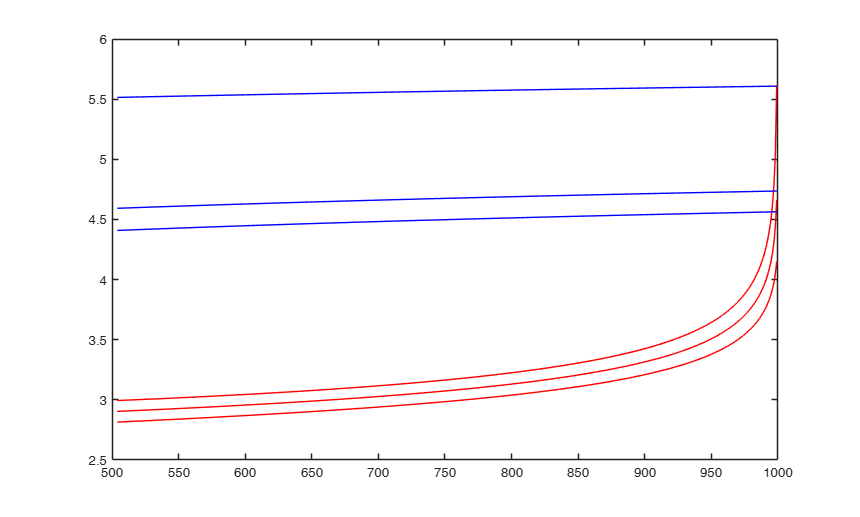# FSMbonfbound

FSMbonfbound computes Bonferroni bounds for each step of the search (in mult analysis)

## Syntax

• Bbound=FSMbonfbound(n,p)example
• Bbound=FSMbonfbound(n,p,Name,Value)example

## Description

 Bbound =FSMbonfbound(n, p) Example using default options.

 Bbound =FSMbonfbound(n, p, Name, Value) Options init and prob.

## Examples

expand all

###Example using default options.

n=1000;
p=5;
init=floor(0.5*(n+p+1))+1;
MMDenv = FSMenvmmd(n,p,'init',init);
Bbound = FSMbonfbound(n,p,'init',init);
figure;
plot(MMDenv(:,1),MMDenv(:,2:end),'r',Bbound(:,1),Bbound(:,2:end),'b');### Options init and prob.

Example using option, init=10 and prob=[0.01 0.05 0.99 0.999]

n=2000;
p=15;
init=100;
prob=[0.95 0.99 0.999];
MMDenv = FSMenvmmd(n,p,'init',init,'prob',prob);
Bbound = FSMbonfbound(n,p,'init',init,'prob',prob);
figure;
plot(MMDenv(:,1),MMDenv(:,2:end),'r',Bbound(:,1),Bbound(:,2:end),'b');

## Related Examples

expand all

### Comparison between chi2 and F distributions.

Example plotting distrib=chi2 and F, init=100 and prob=[0.999].

n=2000;
p=10;
init=100;
prob=[0.99];
MMDenv = FSMenvmmd(n,p,'init',init,'prob',prob);
distrib='chi2';
BboundC = FSMbonfbound(n,p,'init',init,'prob',prob,'distrib',distrib);
distrib='F';
BboundF = FSMbonfbound(n,p,'init',init,'prob',prob,'distrib',distrib);
figure;
plot(MMDenv(:,1),MMDenv(:,2:end),BboundC(:,1),BboundC(:,2:end),BboundF(:,1),BboundF(:,2:end));
legend('Order statistic envelope','Bonferroni Chi2 bound','Bonferroni F bound','Location','best');

## Input Arguments

### n — number of observations. Scalar.

Number of observations on which the envelopes are based.

Data Types: single | double

### p — number of variables. Scalar.

Number of variables on which the envelopes are based.

Data Types: single | double

### Name-Value Pair Arguments

Specify optional comma-separated pairs of Name,Value arguments. Name is the argument name and Value is the corresponding value. Name must appear inside single quotes (' '). You can specify several name and value pair arguments in any order as  Name1,Value1,...,NameN,ValueN.

Example:  'distrib','chi2' , 'init',50 , 'prob',[0.05 0.95] 

### distrib —Reference distribution to use.character.

The statistical distribution used to compute the approximated Bonferroni bounds. Distributions implemented are 'chi2' and 'F' (default).

Example:  'distrib','chi2' 

Data Types: char

### init —Point where to start monitoring required diagnostics.scalar.

Note that if bsb is supplied, init>=length(bsb). If init is not specified it will be set equal to floor(0.5*(n+p+1))+1.

Example:  'init',50 

Data Types: double

### prob —quantiles for which envelopes have to be computed.vector.

Vector containing 1 x k elements .

The default is to produce 1 per cent, 50 per cent and 99 per cent envelopes.

Example:  'prob',[0.05 0.95] 

Data Types: double

## Output Arguments

### Bbound —Bonferroni forward envelopes of mmd.  Matrix

Matrix with n-m0+1 rows and length(prob)+1 columns:

1st col = fwd search index from m0 to n-1, 2nd col = bound for quantile prob, 3rd col = bound for quantile prob, ..., (k+1) col = bound for quantile prob[k].

Atkinson, A.C. and Riani, M. (2006), Distribution theory and simulations for tests of outliers in regression, "Journal of Computational and Graphical Statistics", Vol. 15, pp. 460-476.